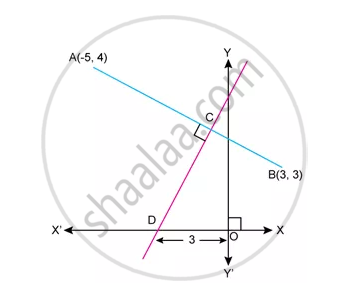Advertisement Remove all ads

# Equation of Ab - Mathematics

Sum

Find equation of ABAdvertisement Remove all ads

#### Solution

Slop Of AB=(3-4)/(3-(-5))=-1/8

∴ Equation of AB is given by

y-4=-1/8(x-(-5))

8y-32=-(x+5)

8y-32=-x-5

x+8y=27

Concept: General Equation of a Line
Is there an error in this question or solution?
Advertisement Remove all ads

#### APPEARS IN

Selina Concise Maths Class 10 ICSE
Chapter 14 Equation of a Line
Exercise 14 (E) | Q 31.1 | Page 204
Advertisement Remove all ads

#### Video TutorialsVIEW ALL 

Advertisement Remove all ads
Share
Notifications

View all notifications

Forgot password?
Course